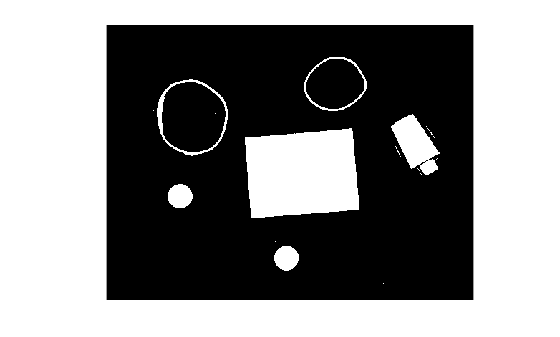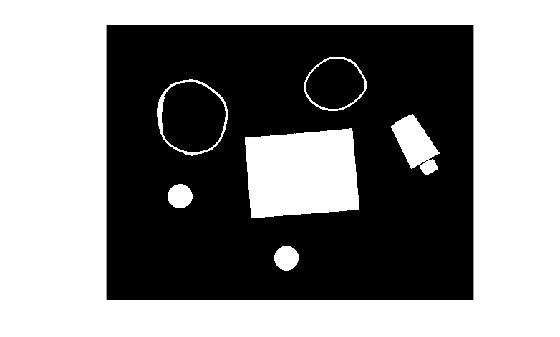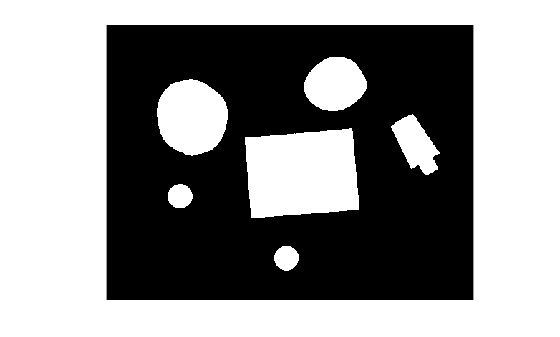Main Content

# Identifying Round Objects

This example shows how to classify objects based on their roundness using `bwboundaries`, a boundary tracing routine.

### Step 1: Read Image

Read in `pills_etc.png`.

```RGB = imread('pillsetc.png'); imshow(RGB)```### Step 2: Threshold the Image

Convert the image to black and white in order to prepare for boundary tracing using `bwboundaries`.

```I = rgb2gray(RGB); bw = imbinarize(I); imshow(bw)```### Step 3: Remove the Noise

Using morphology functions, remove pixels which do not belong to the objects of interest.

Remove all object containing fewer than 30 pixels.

```bw = bwareaopen(bw,30); imshow(bw)```Fill a gap in the pen's cap.

```se = strel('disk',2); bw = imclose(bw,se); imshow(bw)```Fill any holes, so that regionprops can be used to estimate the area enclosed by each of the boundaries

```bw = imfill(bw,'holes'); imshow(bw)```### Step 4: Find the Boundaries

Concentrate only on the exterior boundaries. Option 'noholes' will accelerate the processing by preventing `bwboundaries` from searching for inner contours.

`[B,L] = bwboundaries(bw,'noholes');`

Display the label matrix and draw each boundary.

```imshow(label2rgb(L,@jet,[.5 .5 .5])) hold on for k = 1:length(B) boundary = B{k}; plot(boundary(:,2),boundary(:,1),'w','LineWidth',2) end```### Step 5: Determine which Objects are Round

Estimate each object's area and perimeter. Use these results to form a simple metric indicating the roundness of an object:

`$\mathrm{metric}=\frac{4\pi *\mathrm{area}}{{\mathrm{perimeter}}^{2}}$`

This metric is equal to 1 only for a circle and it is less than one for any other shape. The discrimination process can be controlled by setting an appropriate threshold. In this example use a threshold of 0.94 so that only the pills will be classified as round.

Use `regionprops` to obtain estimates of the area for all of the objects. Notice that the label matrix returned by `bwboundaries` can be reused by `regionprops`.

```stats = regionprops(L,'Area','Centroid'); threshold = 0.94; % loop over the boundaries for k = 1:length(B) % obtain (X,Y) boundary coordinates corresponding to label 'k' boundary = B{k}; % compute a simple estimate of the object's perimeter delta_sq = diff(boundary).^2; perimeter = sum(sqrt(sum(delta_sq,2))); % obtain the area calculation corresponding to label 'k' area = stats(k).Area; % compute the roundness metric metric = 4*pi*area/perimeter^2; % display the results metric_string = sprintf('%2.2f',metric); % mark objects above the threshold with a black circle if metric > threshold centroid = stats(k).Centroid; plot(centroid(1),centroid(2),'ko'); end text(boundary(1,2)-35,boundary(1,1)+13,metric_string,'Color','y',... 'FontSize',14,'FontWeight','bold') end title(['Metrics closer to 1 indicate that ',... 'the object is approximately round'])```Download ebook